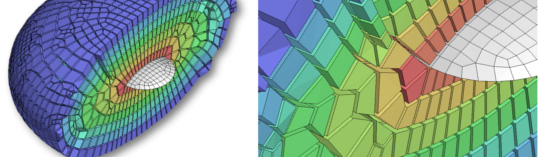## Laboratory of Mathematical & Computational Modelling (LACàN)

You are here: Home / Mathematical & Computational Modelling (LaCàN)

# Laboratory of Mathematical & Computational Modelling (LACàN)Located in Building C2 (2nd floor, Room 210) of UPC-BarcelonaTech's Campus Nord, the Mathematical & Computational Modelling Laboratory (LaCàN) is part of the Mathematical & Computational Modelling section of the Department of Civil & Environmental Engineering. The objective of this laboratory is to develop new mathematical and computational models that allow making science and engineering more quantitative and predictive. One of the central themes common to all our activities is to be pioneers in the mathematical modelling of phenomena using differential equations and their numerical approximation with novel computational methods. Our interdisciplinary research is organized into three research programs interrelated in many respects: computational methods and tools, cell and tissue mechanobiology, and natural and man-made structures and materials.

## Training

The following training activities are carried out in the laboratory:

• PhD theses of the doctoral programs in which the research group participates.
• Final master's theses.
• Final bachelor's theses.
• Preparation of teaching material for bachelor's and master's degree courses.

## Research

In the laboratory, research projects with public and private funding of national and international character are developed, in the following fields of numerical simulation in applied sciences and engineering:

• Computational solid mechanics: large deformations, plasticity, fracture, powder metallurgy, etc.
• Computational mechanics for fluid and wave problems: free surface problems, fluid-structure interaction, compressible and incompressible flows, shallow waters, electromagnetism, acoustics, propagation of pollutants, etc.
• Computational nanomechanics: nanomechanics of biological materials and systems, ferroelectric materials, etc.